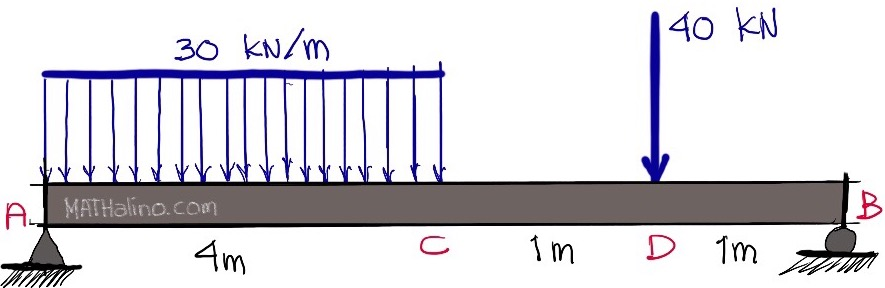# Deflection and rotation of simple beam with uniform and concentrated loadsFor the beam shown below, determine the following:1. Deflection at C
2. Deflection at D
3. Rotation between A and B

• Mathematics inside the configured delimiters is rendered by MathJax. The default math delimiters are $$...$$ and $...$ for displayed mathematics, and $...$ and $...$ for in-line mathematics.